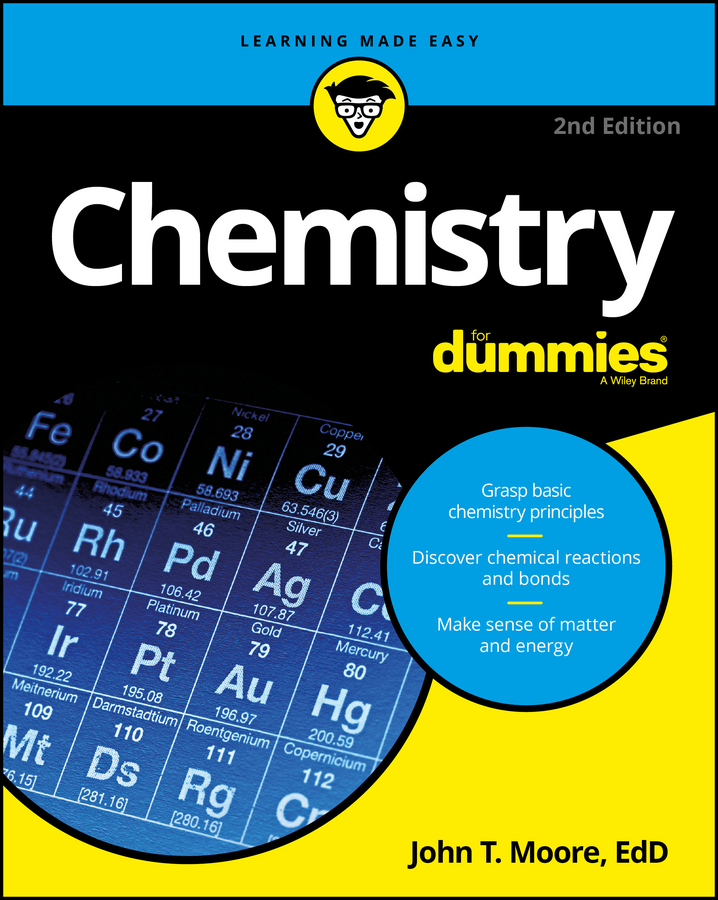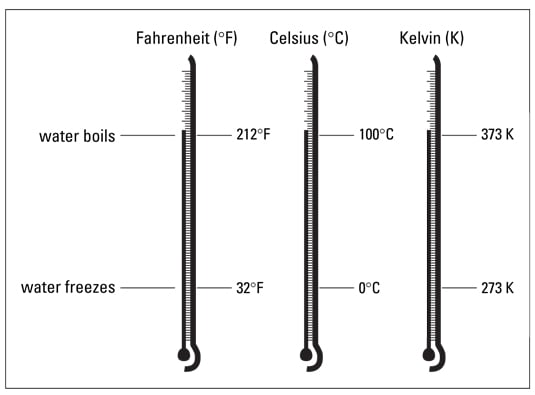##### Chemistry For DummiesYou can measure kinetic energy (energy of motion) with a thermometer. Measuring potential (stored) energy can be a difficult task. The potential energy of a ball stuck up in a tree is related to the mass of the ball and its height above the ground. The potential energy contained in chemical bonds is related to the type of bond and the number of bonds that can potentially break.

## Temperature and temperature scales

When you measure the air temperature in your backyard, you’re really measuring the average kinetic energy of the gas particles in your backyard. The faster those particles are moving, the higher the temperature is.

Now, all of the particles aren’t moving at the same speed. Some are going very fast, and some are going relatively slow, but most are moving at a speed between the two extremes. The temperature reading from your thermometer is related to the average kinetic energy of the particles.

You probably use the Fahrenheit scale to measure temperatures, but most scientists and chemists use either the Celsius (°C) or Kelvin (K) temperature scale. (There’s no degree symbol associated with K.) The following figure compares the three temperature scales using the freezing point and boiling point of water as reference points.Comparison of the Fahrenheit, Celsius, and Kelvin temperature scales
Water boils at 100°C (373 K) and freezes at 0°C (273 K). To get the Kelvin temperature, you take the Celsius temperature and add 273. Mathematically, it looks like this:
K = °C + 273
You may want to know how to convert from Fahrenheit to Celsius (because most of us still think in °F). Here are the equations you need:
°C = 5/9 (°F – 32)
Be sure to subtract 32 from your Fahrenheit temperature before multiplying by 5/9:
°F = 9/5 (°C) + 32
Be sure to multiply your Celsius temperature by 9/5 and then add 32.

Try using these equations to confirm that the normal body temperature of 98.6°F equals 37°C.

## Feel the heat

Heat is not the same as temperature. When you measure the temperature of something, you’re measuring the average kinetic energy of the individual particles. Heat, on the other hand, is a measure of the total amount of energy a substance possesses.

For example, a glass of water and a swimming pool may be the same temperature, but they contain vastly different amounts of heat. It takes much more energy to raise the temperature of a swimming pool 5°C than it does a glass of water, because there’s so much more water in the swimming pool.

The unit of heat in the SI system is the joule (J). Most of us still use the metric unit of heat, the calorie (cal). Here’s the relationship between the two:

1 calorie = 4.184 joule
The calorie is a fairly small amount of heat — the amount it takes to raise the temperature of 1 gram of water 1°C. The kilocalorie (kcal), which is 1,000 calories, is a convenient unit of heat. If you burn a large kitchen match completely, it produces about 1 kilocalorie (1,000 cal) of heat.

When you hear the word calories, you may think about food and counting calories. Food contains energy (heat). The measure of that energy is the nutritional Calorie (which is commonly capitalized), which is really a kilocalorie (kcal). That candy bar you just ate contained 300 nutritional Calories, which is 300 kcal or 300,000 calories.